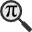## General

Display information for equation id:math.284254.6 on revision:284254

* Page found: Division ring (eq math.284254.6)

(force rerendering)

Cannot find the equation data in the database. Fetching from revision text.

Occurrences on the following pages:

Hash: a51912b521cc149e7fc3df7a5a4daae2

TeX (original user input):

i\in\mathbb{Z}


TeX (checked):

i\in \mathbb {Z}


### LaTeXML (experimental; uses MathML) rendering

MathML (878 B / 342 B) :$i\in{\mathbb{Z}}$
<math xmlns="http://www.w3.org/1998/Math/MathML" id="p1.1.m1.1" class="ltx_Math" alttext="{\displaystyle i\in{\mathbb{Z}}}" display="inline">
<semantics id="p1.1.m1.1a">
<mrow id="p1.1.m1.1.4" xref="p1.1.m1.1.4.cmml">
<mi id="p1.1.m1.1.1" xref="p1.1.m1.1.1.cmml">i</mi>
<mo id="p1.1.m1.1.2" xref="p1.1.m1.1.2.cmml">∈</mo>
<mi id="p1.1.m1.1.3" xref="p1.1.m1.1.3.cmml">ℤ</mi>
</mrow>
<annotation-xml encoding="MathML-Content" id="p1.1.m1.1b">
<apply id="p1.1.m1.1.4.cmml" xref="p1.1.m1.1.4">
<in id="p1.1.m1.1.2.cmml" xref="p1.1.m1.1.2"/>
<ci id="p1.1.m1.1.1.cmml" xref="p1.1.m1.1.1">𝑖</ci>
<ci id="p1.1.m1.1.3.cmml" xref="p1.1.m1.1.3">ℤ</ci>
</apply>
</annotation-xml>
<annotation encoding="application/x-tex" id="p1.1.m1.1c">{\displaystyle i\in{\mathbb{Z}}}</annotation>
</semantics>
[/itex]


SVG (2.499 KB / 1.242 KB) :

### MathML with SVG or PNG fallback (recommended for modern browsers and accessibility tools) rendering

SVG image empty. Force Re-Rendering

SVG (0 B / 8 B) :

PNG (0 B / 8 B) :

$i\in \mathbb {Z}$## Translations to Computer Algebra Systems

### Translation to Maple

In Maple: i in Z

I: You use a typical letter for a constant [the imaginary unit == the principal square root of -1].

We keep it like it is! But you should know that Maple uses I for this constant.

If you want to translate it as a constant, use the corresponding DLMF macro \iunit

i: the imaginary unit == the principal square root of -1 was translated to: i

### Translation to Mathematica

In Mathematica: i \[Element]*Z

I: You use a typical letter for a constant [the imaginary unit == the principal square root of -1].

We keep it like it is! But you should know that Mathematica uses I for this constant.

If you want to translate it as a constant, use the corresponding DLMF macro \iunit

i: the imaginary unit == the principal square root of -1 was translated to: i

## Similar pages

Calculated based on the variables occurring on the entire Division ring page

## Identifiers

• $i$• $\mathbb {Z}$### MathML observations

0results

0results

no statistics present please run the maintenance script ExtractFeatures.php

0 results

0 results